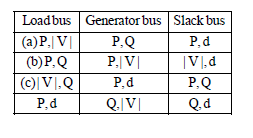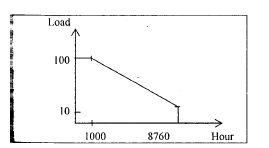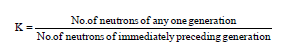# Test: Power Systems - 2

## 25 Questions MCQ Test Electrical Engineering SSC JE (Technical) | Test: Power Systems - 2

Description
Attempt Test: Power Systems - 2 | 25 questions in 50 minutes | Mock test for Electrical Engineering (EE) preparation | Free important questions MCQ to study Electrical Engineering SSC JE (Technical) for Electrical Engineering (EE) Exam | Download free PDF with solutions
QUESTION: 1

Solution:
QUESTION: 2

Solution:
QUESTION: 3

### As per BIS rule the resistance of earth electrode should be measured in ............... weather.

Solution:
QUESTION: 4

A choke is generally used in series with the tube in a fluorescent lamp circuit to

Solution:
QUESTION: 5

In a power house, illumination should be approximately ............

Solution:
QUESTION: 6

Power factor of an alternator driven by constant prime mover input can be changed by changing its

Solution:
QUESTION: 7

The restraining voltage is measured in

Solution:
QUESTION: 8

Where is the draft tube of a hydropower station that is an airtight pipe located ?

Solution:
QUESTION: 9

What is the nominal pH value of water that is to be maintained in a steam raising thermal power station ?

Solution:
QUESTION: 10

The maximum demand of a consumer is 2 kW and the corresponding daily energy consumption is 30 unit. What is the corresponding load factor ?

Solution:
QUESTION: 11

Buses for load flow studies are classified as (i)the load bus, (ii) the generator bus and (iii) the
slack bus The correct combination of the pair of quantities specified having their usual meaning for different buses isSolution:
QUESTION: 12

The impedance value of generator is 0.2 pu on a base value of 11 kV, 50 MVA. The impedance value for a base value of 22 KV, 150 MVA is

Solution:
QUESTION: 13

If the fault current is 2 kA, the relay setting is 50% and the C.T. ratio is 400/5, then the plug setting multiplier of a relay will be

Solution:
QUESTION: 14

A power plant has a maximum demand of 15 MW. The load factor is 50% and the plant factor is 40%. The operating reserve is

Solution:
QUESTION: 15

The load curve of system is shown in the figure.The load factor of the system is

Solution:
QUESTION: 16

Galloping in transmission line conductors arises generally due to

Solution:

Galloping is a low frequency, large amplitude, wind-induced vibration of both single and bundle overhead conductors, with a single or a few loops of standing waves per span. It is caused by moderately strong, steady crosswind acting upon an asymmetrically- iced conductor surface.

QUESTION: 17

A thermal generating station has an installed capacity of 15 MW and supplies a daily load of 10 MW for 12 hours and 5 MW of remaining 12 hours. The plant capacity factor for this station is

Solution:

Plant capacity factor =

average energy produced/maximum possible energy based on installed capacity

= 10 x12 + 5 x 12 / 24 x 15 = 0.5

QUESTION: 18

Compared to Gauss-seidel method, Newton- Raphson method takes

Solution:
QUESTION: 19

The operation of a nuclear reactor is controlled by controlling multipliction factor (K) defined asThe power -level of the reactor can be increased by

Solution:
QUESTION: 20

Non-metallic conduits used in internal wiring are generally made of

Solution:
QUESTION: 21

PVC cables are usually used for

Solution:
QUESTION: 22

The rating of fuse wire is always expressed in

Solution:
QUESTION: 23

Protection by fuses is generally not used beyond

Solution:
QUESTION: 24

Energy meter, for connecting, has ––––––– terminals.

Solution:

Explanation : An energy meter usually has four terminals. A pair of terminals for the current coil and another pair for the Voltage coil (a.k.a pressure coil). As their name suggests, current coil is in series with the line and or produces magnetic field according to the current flow.

Whereas Voltage coil is in parallel with the Load and it produces magnetic field according to the voltage drop across the Load.

These two fields combine to produce a torque which rotates the aluminum disc on a conventional Energy meter.

QUESTION: 25

The positive sequence current for a L-L fault of a 2 kV system is 1400 A, and corresponding current for a L-L-G fault is 2220 A. The zero sequence impedance of the system is

Solution:Use Code STAYHOME200 and get INR 200 additional OFF Use Coupon Code